# 8  Case Study in Data Reduction

Recall that the aim of data reduction is to reduce (without using the outcome) the number of parameters needed in the outcome model. The following case study illustrates these techniques:

1. redundancy analysis;
2. variable clustering;
3. data reduction using principal component analysis (PCA), sparse PCA, and pre-transformations;
4. restricted cubic spline fitting using ordinary least squares, in the context of scaling; and
5. scaling/variable transformations using canonical variates and nonparametric additive regression.

## 8.1 Data

Consider the 506-patient prostate cancer dataset from Byar & Green (1980). The data are listed in [Andrews & Herzberg (1985); Table 46] and are available at https://hbiostat.org/data. These data were from a randomized trial comparing four treatments for stage 3 and 4 prostate cancer, with almost equal numbers of patients on placebo and each of three doses of estrogen. Four patients had missing values on all of the following variables: wt, pf, hx, sbp, dbp, ekg, hg, bm; two of these patients were also missing sz. These patients are excluded from consideration. The ultimate goal of an analysis of the dataset might be to discover patterns in survival or to do an analysis of covariance to assess the effect of treatment while adjusting for patient heterogeneity. See Chapter Chapter 21 for such analyses. The data reductions developed here are general and can be used for a variety of dependent variables.

The variable names, labels, and a summary of the data are printed below. Because of extreme skewness, ap is log-transformed for the purpose of making its spike histogram.

Code
require(Hmisc)
# Convert an old date format to R format
prostate$sdate <- as.Date(prostate$sdate)
d <- describe(prostate[2:17],
trans=list(ap=list('log', log, exp)))
sparkline::sparkline(0)   # load sparkline javascript dependencies
Code
maketabs(print(d, 'both'), initblank=TRUE, wide=TRUE)
Variable Label n Missing Distinct Info Mean Gini |Δ| prostate[2:17] Descriptives 9 Continous Variables of 16 Variables, 502 Observations dtime Months of Follow-up 502 0 76 1.000 36.13 26.89 1.05 5.00 14.25 34.00 57.75 67.00 71.00 age Age in Years 501 1 41 0.996 71.46 7.497 56 60 70 73 76 78 80 wt Weight Index = wt(kg)-ht(cm)+200 500 2 67 0.999 99.03 14.93 77.95 82.90 90.00 98.00 107.00 116.00 123.00 sbp Systolic Blood Pressure/10 502 0 18 0.980 14.35 2.596 11 12 13 14 16 17 18 dbp Diastolic Blood Pressure/10 502 0 12 0.945 8.149 1.553 6 6 7 8 9 10 10 hg Serum Hemoglobin (g/100ml) 502 0 91 1.000 13.45 2.16 10.2 10.7 12.3 13.7 14.7 15.8 16.4 sz Size of Primary Tumor (cm^2) 497 5 55 0.998 14.63 13.05 2.0 3.0 5.0 11.0 21.0 32.0 39.2 sg Combined Index of Stage and Hist. Grade 491 11 11 0.959 10.31 2.245 8 8 9 10 11 13 13 ap Serum Prostatic Acid Phosphatase 502 0 128 0.996 12.18 21.71 0.300 0.300 0.500 0.700 2.975 21.689 38.470
Variable Label n Missing Distinct Info Sum Mean Gini |Δ| prostate[2:17] Descriptives 7 Categorical Variables of 16 Variables, 502 Observations stage Stage 502 0 2 0.733 3.424 0.4895 rx 502 0 4 status 502 0 10 pf 502 0 4 hx History of Cardiovascular Disease 502 0 2 0.733 213 0.4243 0.4895 ekg 494 8 7 bm Bone Metastases 502 0 2 0.410 82 0.1633 0.2739

stage is defined by ap as well as X-ray results. Of the patients in stage 3, 0.92 have ap $$\leq$$ 0.8. Of those in stage 4, 0.93 have ap > 0.8. Since stage can be predicted almost certainly from ap, we do not consider stage in some of the analyses.

## 8.2 How Many Parameters Can Be Estimated?

There are 354 deaths among the 502 patients. If predicting survival time were of major interest, we could develop a reliable model if no more than about $$354/15 = 24$$ parameters were examined against $$Y$$ in unpenalized modeling. Suppose that a full model with no interactions is fitted and that linearity is not assumed for any continuous predictors. Assuming age is almost linear, we could fit a restricted cubic spline function with three knots. For the other continuous variables, let us use five knots. For categorical predictors, the maximum number of degrees of freedom needed would be one fewer than the number of categories. For pf we could lump the last two categories since the last category has only 2 patients. Likewise, we could combine the last two levels of ekg. Table 8.1 lists candidate predictors with the maximum number of parameters we consider for each.

Table 8.1: Degrees of fredom needed for predictors
Predictor: rx age wt pf hx sbp dbp ekg hg sz sg ap bm
Number of Parameters: 3 2 4 2 1 4 4 5 4 4 4 4 1

## 8.3 Redundancy Analysis

As described in Section Section 4.7.1, it is occasionally useful to do a rigorous redundancy analysis on a set of potential predictors. Let us run the algorithm discussed there, on the set of predictors we are considering. We will use a low threshold (0.3) for $$R^2$$ for demonstration purposes.

Code
# Allow only 1 d.f. for three of the predictors
prostate <-
transform(prostate,
ekg.norm = 1*(ekg %in% c("normal","benign")),
rxn = as.numeric(rx),
pfn = as.numeric(pf))
# Force pfn, rxn to be linear because of difficulty of placing
# knots with so many ties in the data
# Note: all incomplete cases are deleted (inefficient)
redun(~ stage + I(rxn) + age + wt + I(pfn) + hx +
sbp + dbp + ekg.norm + hg + sz + sg + ap + bm,
r2=.3, type='adjusted', data=prostate)

Redundancy Analysis

~stage + I(rxn) + age + wt + I(pfn) + hx + sbp + dbp + ekg.norm +
hg + sz + sg + ap + bm

n: 483  p: 14   nk: 3

Number of NAs:   19
Frequencies of Missing Values Due to Each Variable
stage   I(rxn)      age       wt   I(pfn)       hx      sbp      dbp
0        0        1        2        0        0        0        0
ekg.norm       hg       sz       sg       ap       bm
0        0        5       11        0        0

Transformation of target variables forced to be linear

R^2 with which each variable can be predicted from all other variables:

stage   I(rxn)      age       wt   I(pfn)       hx      sbp      dbp
0.658    0.000    0.073    0.111    0.156    0.062    0.452    0.417
ekg.norm       hg       sz       sg       ap       bm
0.055    0.146    0.192    0.540    0.147    0.391

Rendundant variables:

stage sbp bm sg

Predicted from variables:

I(rxn) age wt I(pfn) hx dbp ekg.norm hg sz ap

Variable Deleted   R^2 R^2 after later deletions
1            stage 0.658         0.658 0.646 0.494
2              sbp 0.452               0.453 0.455
3               bm 0.374                     0.367
4               sg 0.342                          

By any reasonable criterion on $$R^2$$, none of the predictors is redundant. stage can be predicted with an $$R^{2} = 0.658$$ from the other 13 variables, but only with $$R^{2} = 0.493$$ after deletion of 3 variables later declared to be “redundant.”

## 8.4 Variable Clustering

From Table 8.1, the total number of parameters is 42, so some data reduction should be considered. We resist the temptation to take the “easy way out” using stepwise variable selection so that we can achieve a more stable modeling process 1 and obtain unbiased standard errors. Before using a variable clustering procedure, note that ap is extremely skewed. To handle skewness, we use Spearman rank correlations for continuous variables (later we transform each variable using transcan, which will allow ordinary correlation coefficients to be used). After classifying ekg as “normal/benign” versus everything else, the Spearman correlations are plotted below.

• 1 Sauerbrei & Schumacher (1992) used the bootstrap to demonstrate the variability of a standard variable selection procedure for the prostate cancer dataset.

• Code
x <- with(prostate,
cbind(stage, rx, age, wt, pf, hx, sbp, dbp,
ekg.norm, hg, sz, sg, ap, bm))
# If no missing data, could use cor(apply(x, 2, rank))
r <- rcorr(x, type="spearman")$r # rcorr in Hmisc maxabsr <- max(abs(r[row(r) != col(r)])) Code plotCorrM(r)[] # An Hmisc function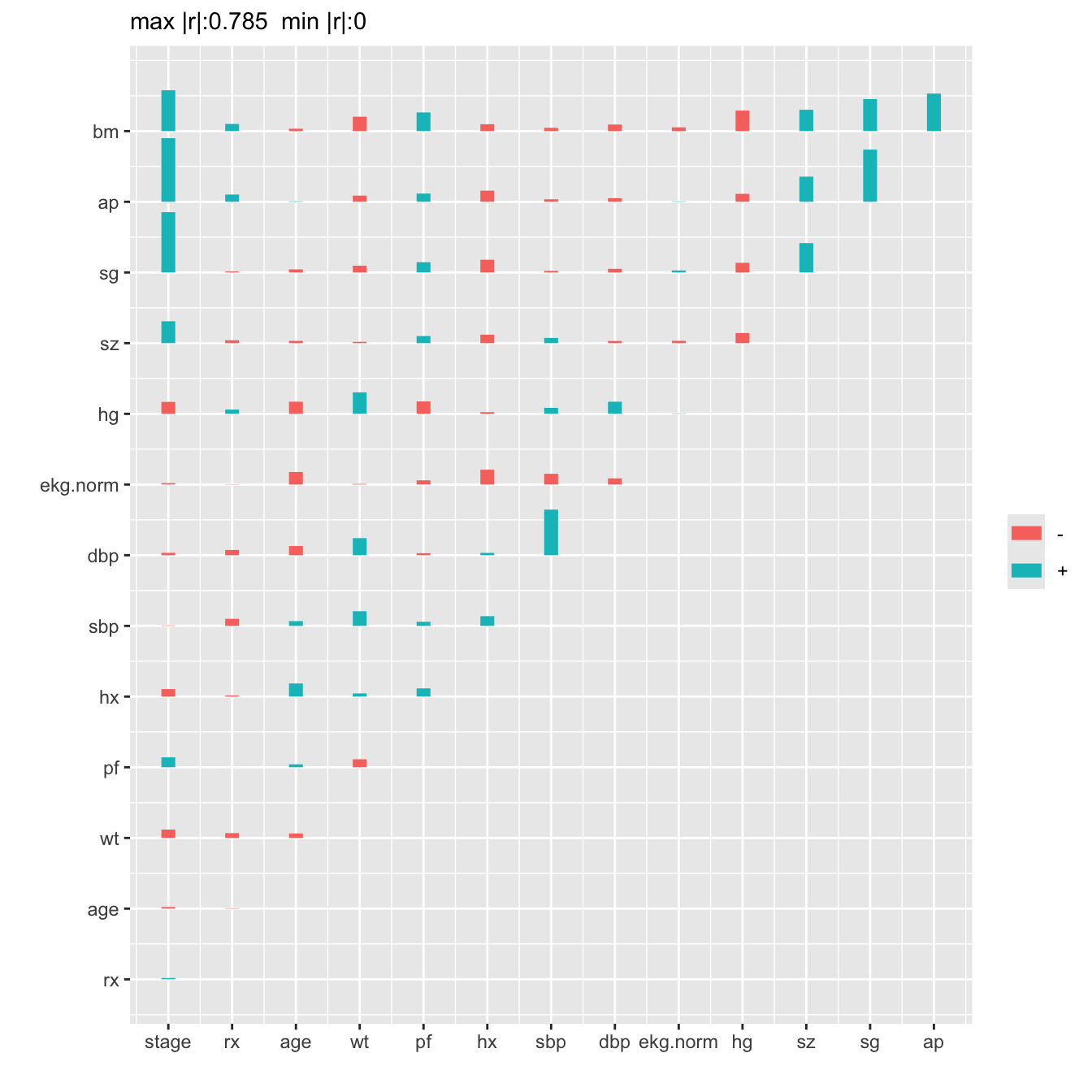Figure 8.1: Matrix of Spearman $$\rho$$ rank correlation coefficients between predictors. Horizontal gray scale lines correspond to $$\rho=0$$. The tallest bar corresponds to $$|\rho|=0.785$$. We perform a hierarchical cluster analysis based on a similarity matrix that contains pairwise Hoeffding $$D$$ statistics $$D$$ will detect nonmonotonic associations. Code vc <- varclus(~ stage + rxn + age + wt + pfn + hx + sbp + dbp + ekg.norm + hg + sz + sg + ap + bm, sim='hoeffding', data=prostate) plot(vc)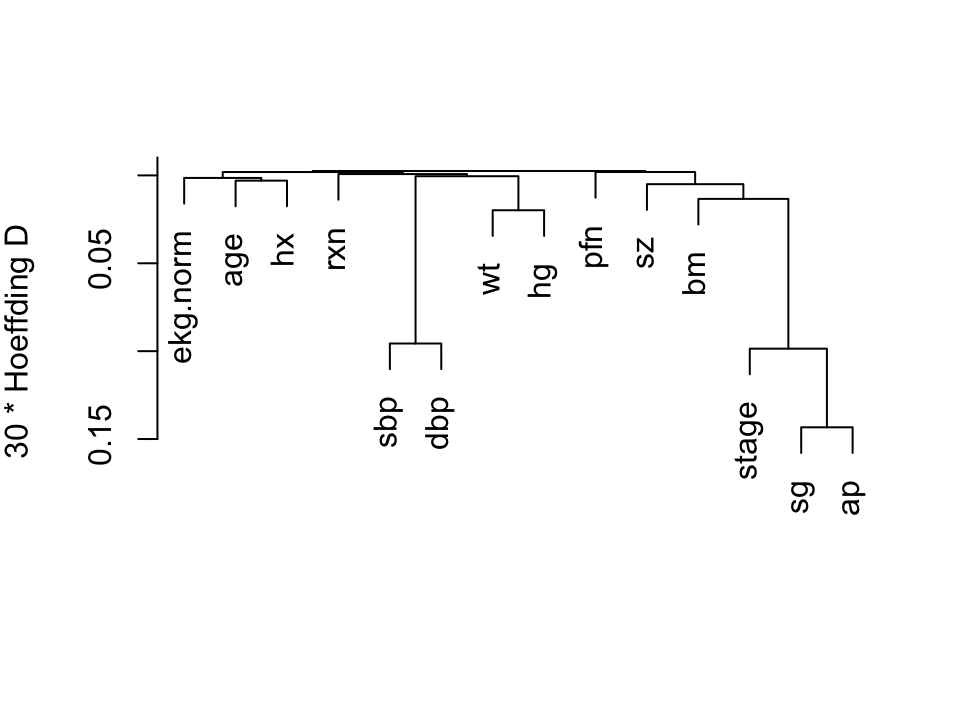Figure 8.2: Hierarchical clustering using Hoeffding’s $$D$$ as a similarity measure. Dummy variables were used for the categorical variable ekg. Some of the dummy variables cluster together since they are by definition negatively correlated. We combine sbp and dbp, and tentatively combine ap, sg, sz, and bm. ## 8.5 Transformation and Single Imputation Using transcan Now we turn to the scoring of the predictors to potentially reduce the number of regression parameters that are needed later by doing away with the need for nonlinear terms and multiple dummy variables. The R Hmisc package transcan function defaults to using a maximum generalized variance method that incorporates canonical variates to optimally transform both sides of a multiple regression model. Each predictor is treated in turn as a variable being predicted, and all variables are expanded into restricted cubic splines (for continuous variables) or dummy variables (for categorical ones). Code spar(bot=1) # Combine 2 levels of ekg (one had freq. 1) levels(prostate$ekg)[levels(prostate$ekg) %in% c('old MI', 'recent MI')] <- 'MI' prostate$pf.coded <- as.integer(prostate$pf) # make a numeric version; combine last 2 levels of original levels(prostate$pf) <- levels(prostate$pf)[c(1,2,3,3)] ptrans <- transcan(~ sz + sg + ap + sbp + dbp + age + wt + hg + ekg + pf + bm + hx, imputed=TRUE, transformed=TRUE, trantab=TRUE, pl=FALSE, show.na=TRUE, data=prostate, frac=.1, pr=FALSE) summary(ptrans, digits=4) transcan(x = ~sz + sg + ap + sbp + dbp + age + wt + hg + ekg + pf + bm + hx, imputed = TRUE, trantab = TRUE, transformed = TRUE, pr = FALSE, pl = FALSE, show.na = TRUE, data = prostate, frac = 0.1) Iterations: 8 R-squared achieved in predicting each variable: sz sg ap sbp dbp age wt hg ekg pf bm hx 0.207 0.556 0.573 0.498 0.485 0.095 0.122 0.158 0.092 0.113 0.349 0.108 Adjusted R-squared: sz sg ap sbp dbp age wt hg ekg pf bm hx 0.180 0.541 0.559 0.481 0.468 0.065 0.093 0.129 0.059 0.086 0.331 0.083 Coefficients of canonical variates for predicting each (row) variable sz sg ap sbp dbp age wt hg ekg pf bm hx sz 0.66 0.20 0.33 0.33 -0.01 -0.01 0.11 0.11 0.03 -0.36 0.34 sg 0.23 0.84 0.08 0.07 -0.02 0.01 -0.01 -0.07 0.02 -0.20 0.14 ap 0.07 0.80 -0.11 -0.05 0.03 -0.02 0.01 0.01 0.00 -0.83 -0.03 sbp 0.13 0.10 -0.14 -0.94 0.14 -0.09 0.03 0.10 0.10 -0.03 -0.14 dbp 0.13 0.09 -0.06 -0.98 0.14 0.07 0.05 0.03 0.04 0.03 -0.01 age -0.02 -0.06 0.18 0.58 0.57 0.14 0.46 0.43 -0.03 1.05 -0.76 wt -0.02 0.06 -0.08 -0.31 0.23 0.12 0.51 -0.06 0.21 -1.09 0.27 hg 0.13 -0.02 0.03 0.09 0.15 0.33 0.43 -0.02 0.24 -1.53 -0.12 ekg 0.20 -0.38 0.10 0.42 0.12 0.41 -0.04 -0.04 0.15 -0.42 -1.23 pf 0.04 0.08 0.02 0.36 0.14 -0.03 0.22 0.29 0.13 -1.75 -0.46 bm -0.02 -0.03 -0.13 0.00 0.00 0.03 -0.04 -0.06 -0.01 -0.06 -0.02 hx 0.04 0.05 -0.01 -0.04 0.00 -0.06 0.02 -0.01 -0.09 -0.04 -0.05 Summary of imputed values  Starting estimates for imputed values: sz sg ap sbp dbp age wt hg ekg pf bm hx 11.0 10.0 0.7 14.0 8.0 73.0 98.0 13.7 1.0 1.0 0.0 0.0  Code ggplot(ptrans, scale=TRUE) + theme(axis.text.x=element_text(size=6))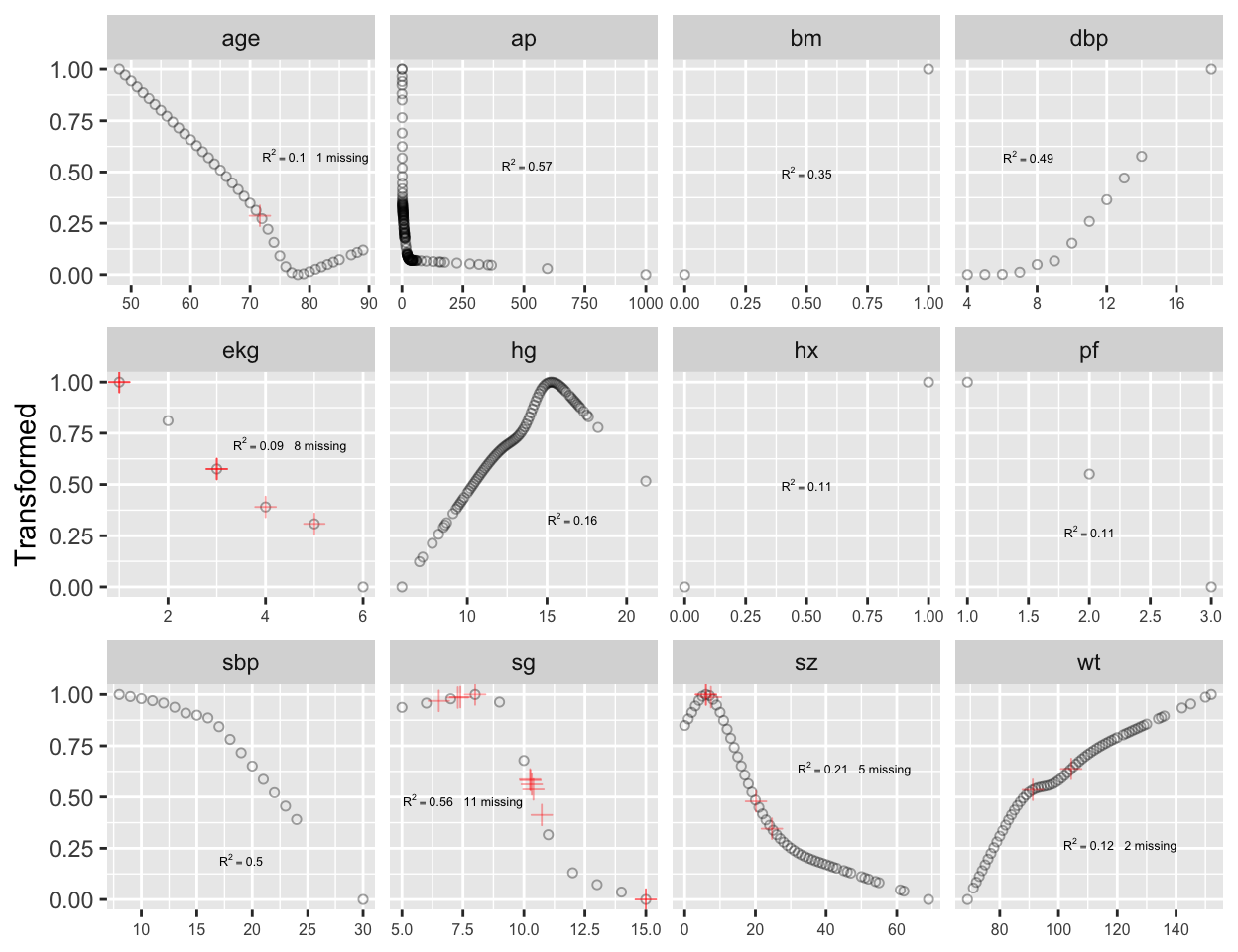Figure 8.3: Simultaneous transformation and single imputation of all candidate predictors using transcan. Imputed values are shown as red plus signs. Transformed values are arbitrarily scaled to $$[0,1]$$. Note that at face value the transformation of ap was derived in a circular manner, since the combined index of stage and histologic grade, sg, uses in its stage component a cutoff on ap. However, if sg is omitted from consideration, the resulting transformation for ap does not change appreciably. Note that bm and hx are represented as binary variables, so their coefficients in the table of canonical variable coefficients are on a different scale. For the variables that were actually transformed, the coefficients are for standardized transformed variables (mean 0, variance 1). From examining the $$R^2$$s, age, wt, ekg, pf, and hx are not strongly related to other variables. Imputations for age, wt, ekg are thus relying more on the median or modal values from the marginal distributions. From the coefficients of first (standardized) canonical variates, sbp is predicted almost solely from dbp; bm is predicted mainly from ap, hg, and pf. 2 • 2 Schemper & Heinze (1997) used logistic models to impute dichotomizations of the predictors for this dataset. • ## 8.6 Data Reduction Using Principal Components The first PC, PC$$_{1}$$, is the linear combination of standardized variables having maximum variance. PC$$_{2}$$ is the linear combination of predictors having the second largest variance such that PC$$_{2}$$ is orthogonal to (uncorrelated with) PC$$_{1}$$. If there are $$p$$ raw variables, the first $$k$$ PCs, where $$k < p$$, will explain only part of the variation in the whole system of $$p$$ variables unless one or more of the original variables is exactly a linear combination of the remaining variables. Note that it is common to scale and center variables to have mean zero and variance 1 before computing PCs. The response variable (here, time until death due to any cause) is not examined during data reduction, so that if PCs are selected by variance explained in the $$X$$-space and not by variation explained in $$Y$$, one needn’t correct for model uncertainty or multiple comparisons. PCA results in data reduction when the analyst uses only a subset of the $$p$$ possible PCs in predicting $$Y$$. This is called incomplete principal component regression. When one sequentially enters PCs into a predictive model in a strict pre-specified order (i.e., by descending amounts of variance explained for the system of $$p$$ variables), model uncertainty requiring bootstrap adjustment is minimized. In contrast, model uncertainty associated with stepwise regression (driven by associations with $$Y$$) is massive. For the prostate dataset, consider PCs on raw candidate predictors, expanding polytomous factors using indicator variables. The R function princmp is used, after singly imputing missing raw values using transcan’s optimal additive nonlinear models. In this series of analyses we ignore the treatment variable, rx. princmp calls the built-in princomp function. princmp assists with interpretation of PCs. Code spar(top=1, ps=7) # Impute all missing values in all variables given to transcan imputed <- impute(ptrans, data=prostate, list.out=TRUE)  Imputed missing values with the following frequencies and stored them in variables with their original names: sz sg age wt ekg 5 11 1 2 8  Code imputed <- as.data.frame(imputed) # Compute principal components on imputed data. princmp will expand # the categorical variable EKG into indicator variables. # Use correlation matrix pfn <- prostate$pfn
prin.raw <- princmp(~ sz + sg + ap + sbp + dbp + age +
wt + hg + ekg + pfn + bm + hx,
k=16, sw=TRUE, data=imputed)
prin.raw
Principal Components Analysis

Stepwise Approximations to PCs With Cumulative R^2

PC 1
bm (0.486) + dbp (0.643) + sg (0.743) + hg (0.814) + ap (0.871)

PC 2
sbp (0.514) + ekgheart strain (0.648) + sg (0.754) + dbp (0.836) + pfn
(0.888)

PC 3
ekgMI (0.376) + hx (0.63) + age (0.795) + pfn (0.894) + hg (0.942)

PC 4
ekgheart strain (0.453) + ekgrhythmic disturb & electrolyte ch (0.631)
+ sbp (0.754) + sz (0.809) + ekgheart block or conduction def (0.853)

PC 5
age (0.198) + ekgbenign (0.37) + pfn (0.516) + ekgMI (0.624) + wt
(0.718)
Code
plot(prin.raw, ylim=c(0,4), offset=1)
prin.trans <- princmp(ptrans$transformed, k=12) prin.trans Principal Components Analysis Code plot(prin.trans, add=TRUE, col='red', offset=-1.3)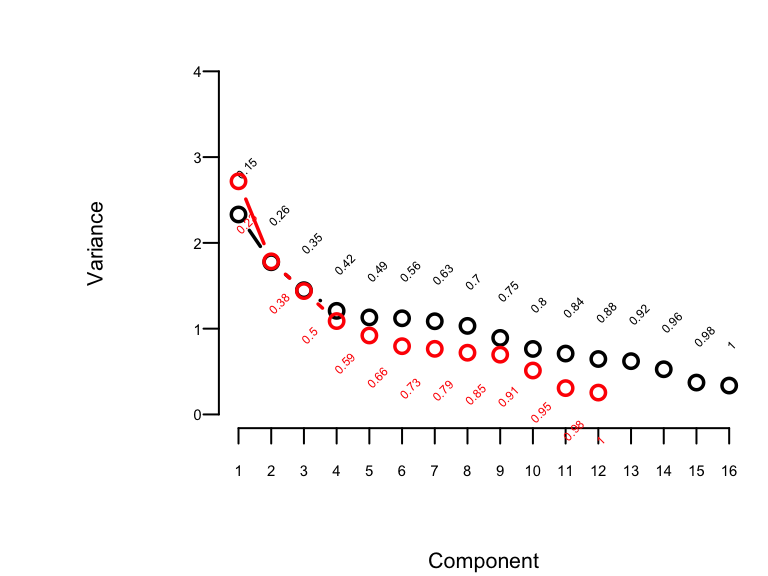Figure 8.4: Variance of the system of raw predictors (black) explained by individual principal components (lines) along with cumulative proportion of variance explained (text), and variance explained by components computed on transcan-transformed variables (red) The plot shown in Figure 8.4 is called a “scree” plot [Jolliffe (2010); pp. 96–99, 104, 106]. It shows the variation explained by the first $$k$$ principal components as $$k$$ increases all the way to 16 parameters (no data reduction). It requires 10 of the 16 possible components to explain $$> 0.8$$ of the variance, and the first 5 components explain $$0.49$$ of the variance of the system. Two of the 16 dimensions are almost totally redundant. Code plot(prin.raw, 'loadings', k=6)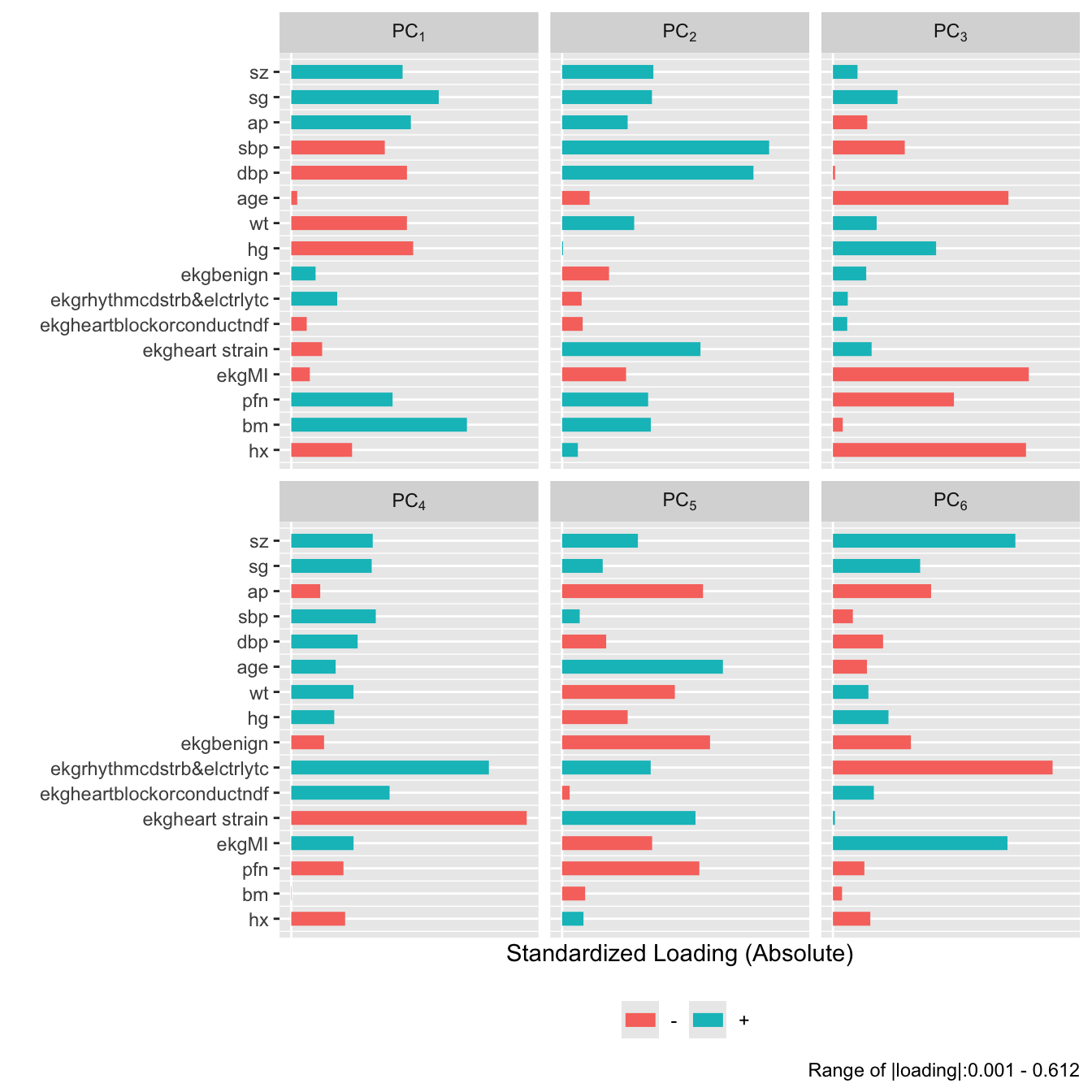Code plot(prin.trans, 'loadings', k=6)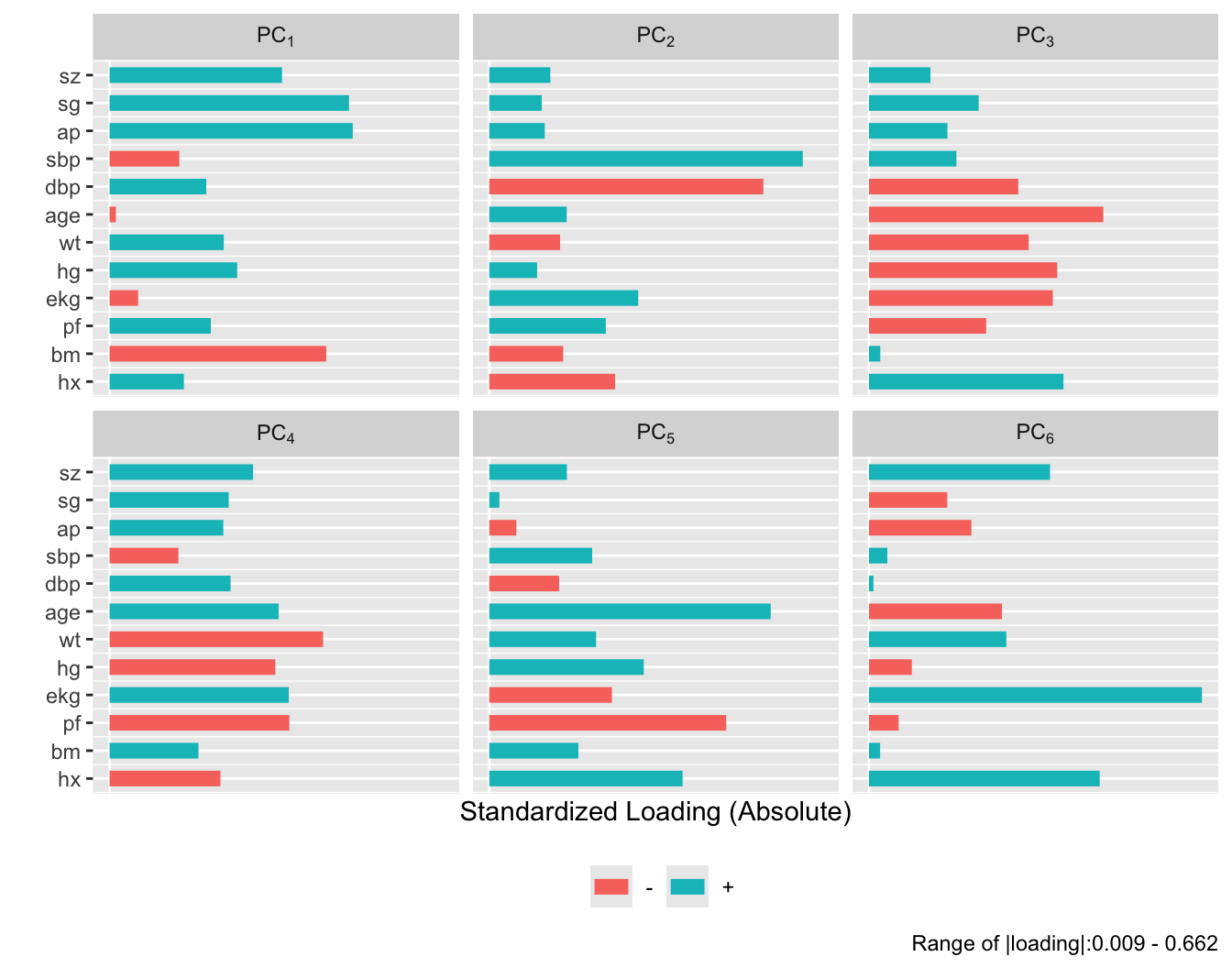After repeating this process when transforming all predictors via transcan, we have only 12 degrees of freedom for the 12 predictors. The variance explained is depicted in Figure 8.4 in red. It requires at least 8 of the 12 possible components to explain $$\geq 0.8$$ of the variance, and the first 5 components explain $$0.66$$ of the variance as opposed to $$0.49$$ for untransformed variables. Let us see how the PCs “explain” the times until death using the Cox regression function from rms, cph, described in Chapter 20. In what follows we vary the number of components used in the Cox models from 1 to all 16, computing the AIC for each model. AIC is related to model log likelihood penalized for number of parameters estimated, and lower is better. For reference, the AIC of the model using all of the original predictors, and the AIC of a full additive spline model are shown as horizontal lines. Code require(rms) spar(bty='l') S <- with(prostate, Surv(dtime, status != "alive")) # two-column response var. pcs <- prin.raw$scores         # pick off all PCs
aic <- numeric(16)
for(i in 1:16) {
ps <- pcs[,1:i]
aic[i] <- AIC(cph(S ~ ps))
}
plot(1:16, aic, xlab='Number of Components Used',
ylab='AIC', type='l', ylim=c(3950,4000))
f <- cph(S ~ sz + sg + log(ap) + sbp + dbp + age + wt + hg +
ekg + pf + bm + hx, data=imputed)
abline(h=AIC(f), col='blue')
## The following model in the 2nd edition no longer converges
# f <- cph(S ~ rcs(sz,5) + rcs(sg,5) + rcs(log(ap),5) +
#          rcs(sbp,5) + rcs(dbp,5) + rcs(age,3) + rcs(wt,5) +
#          rcs(hg,5) + ekg + pf + bm + hx,
#          tol=1e-14, data=imputed)
f <- cph(S ~ rcs(sz,4) + rcs(sg,4) + rcs(log(ap),5) +
rcs(sbp,4) + rcs(dbp,4) + rcs(age,3) + rcs(wt,4) +
rcs(hg,4) + ekg + pf + bm + hx,
tol=1e-14, data=imputed)
abline(h=AIC(f), col='blue', lty=2)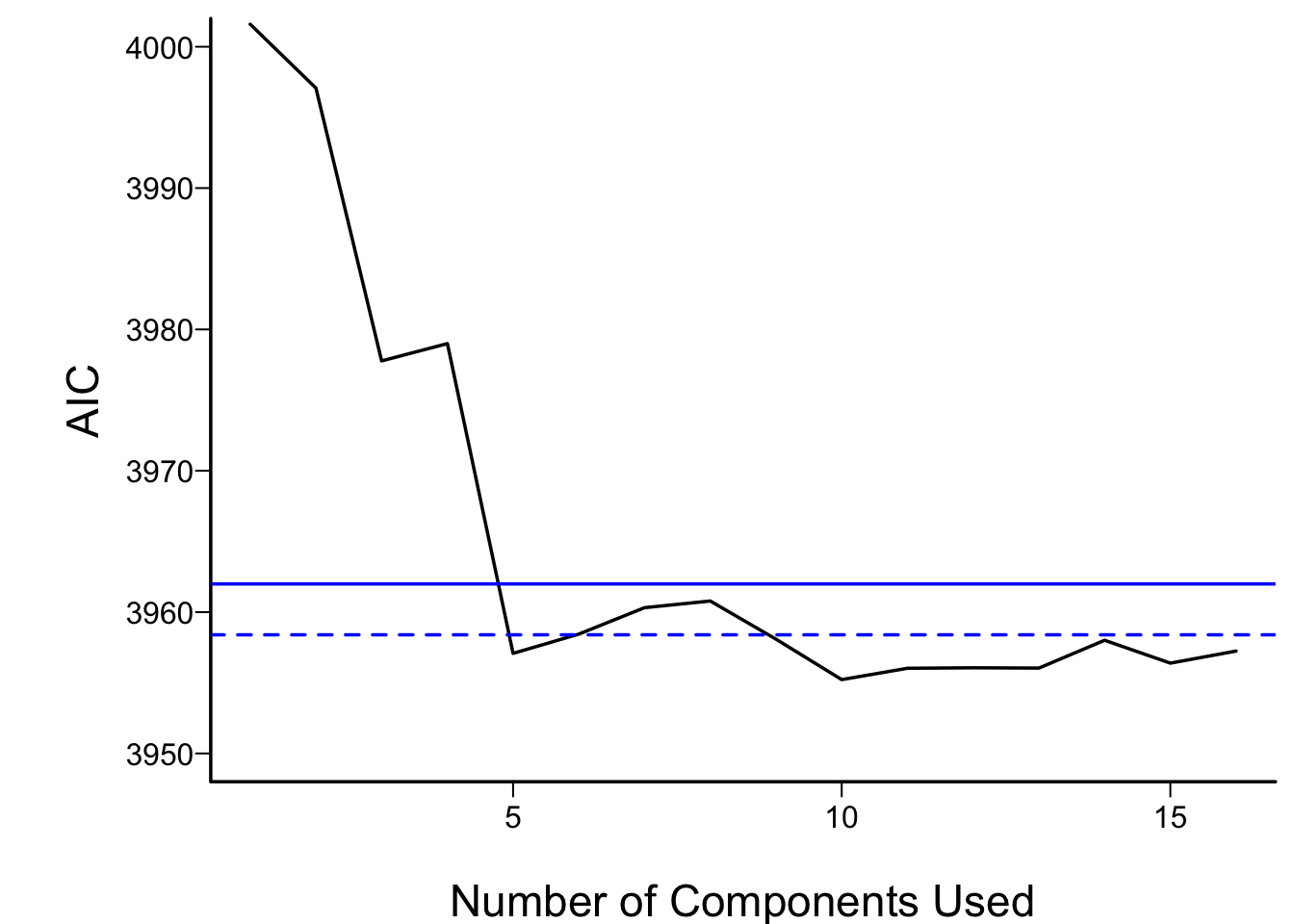Figure 8.5: AIC of Cox models fitted with progressively more principal components. The solid blue line depicts the AIC of the model with all original covariates. The dotted blue line is positioned at the AIC of the full spline model.

For the money, the first 5 components adequately summarizes all variables, if linearly transformed, and the full linear model is no better than this. The model allowing all continuous predictors to be nonlinear is not worth its added degrees of freedom.

Next check the performance of a model derived from cluster scores of transformed variables.

Code
# Compute PC1 on a subset of transcan-transformed predictors
pco <- function(v) {
f <- princmp(ptrans$transformed[,v]) print(f) vars <- attr(f, 'results')$vars
cat('Fraction of variance explained by PC1:',
round(vars / sum(vars),2), '\n')
f$scores[,1] } tumor <- pco(c('sz','sg','ap','bm')) Principal Components Analysis Fraction of variance explained by PC1:  Code bp <- pco(c('sbp','dbp')) Principal Components Analysis Fraction of variance explained by PC1:  Code cardiac <- pco(c('hx','ekg')) Principal Components Analysis Fraction of variance explained by PC1:  Code # Get transformed individual variables that are not clustered other <- ptrans$transformed[,c('hg','age','pf','wt')]
f <- cph(S ~ tumor + bp + cardiac + other)  # other is matrix
AIC(f)
 3954.393
Code
print(f, long=FALSE, title='')
Model Tests Discrimination
Indexes
Obs 502 LR χ2 81.11 R2 0.149
Events 354 d.f. 7 R27,502 0.137
Center 0 Pr(>χ2) 0.0000 R27,354 0.189
Score χ2 86.81 Dxy 0.286
Pr(>χ2) 0.0000
β S.E. Wald Z Pr(>|Z|)
tumor  -0.1723  0.0367 -4.69 <0.0001
bp   0.0251  0.0424 0.59 0.5528
cardiac   0.2513  0.0516 4.87 <0.0001
hg  -0.1407  0.0554 -2.54 0.0111
age  -0.1034  0.0579 -1.79 0.0739
pf  -0.0933  0.0487 -1.92 0.0551
wt  -0.0910  0.0555 -1.64 0.1012

The tumor and cardiac clusters seem to dominate prediction of mortality, and the AIC of the model built from cluster scores of transformed variables compares favorably with other models (Figure 8.5).

### 8.6.1 Sparse Principal Components

A disadvantage of principal components is that every predictor receives a nonzero weight for every component, so many coefficients are involved even through the effective degrees of freedom with respect to the response model are reduced. Sparse principal components uses a penalty function to reduce the magnitude of the loadings variables receive in the components. If an L1 penalty is used (as with the lasso, some loadings are shrunk to zero, resulting in some simplicity. Sparse principal components combines some elements of variable clustering, scoring of variables within clusters, and redundancy analysis.

Filzmoser et al. (2012) have written a nice R package pcaPP for doing sparse PC analysis.3 The following example uses the prostate data again. To allow for nonlinear transformations and to score the ekg variable in the prostate dataset down to a scalar, we use the transcan-transformed predictors as inputs.

• 3 The spcr package is another sparse PC package that should also be considered.

• Code
s <- princmp(ptrans$transformed, k=10, method='sparse', sw=TRUE, nvmax=3) s Sparse Principal Components Analysis Stepwise Approximations to PCs With Cumulative R^2 PC 1 sg (0.83) + ap (0.962) + sz (0.992) PC 2 sbp (0.836) + dbp (1) PC 3 ekg (1) PC 4 age (1) PC 5 hx (1) Code plot(s)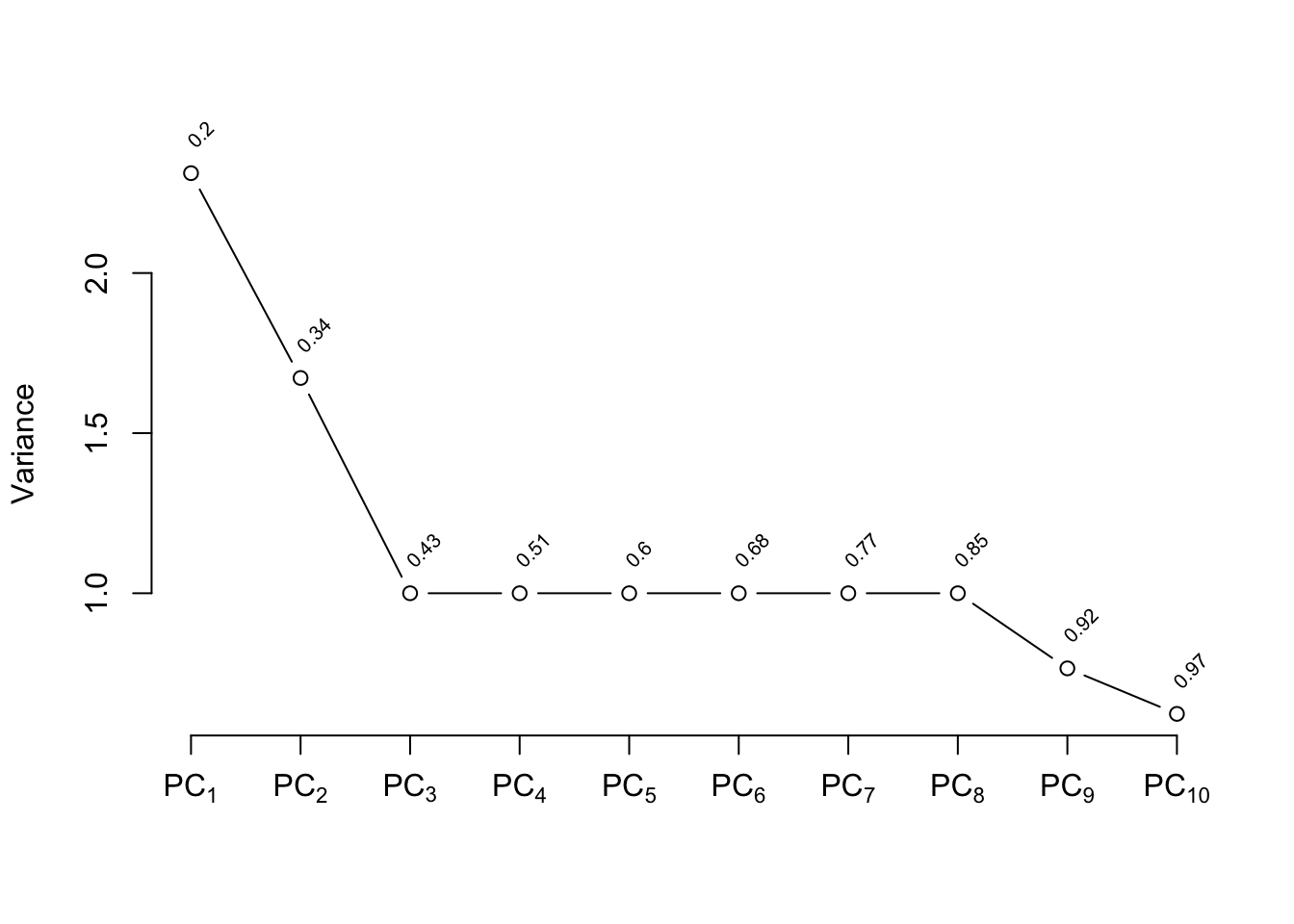Figure 8.6: Variance explained by individual sparse principal components (lines) along with cumulative proportion of variance explained (text) Code plot(s, 'loadings', nrow=1)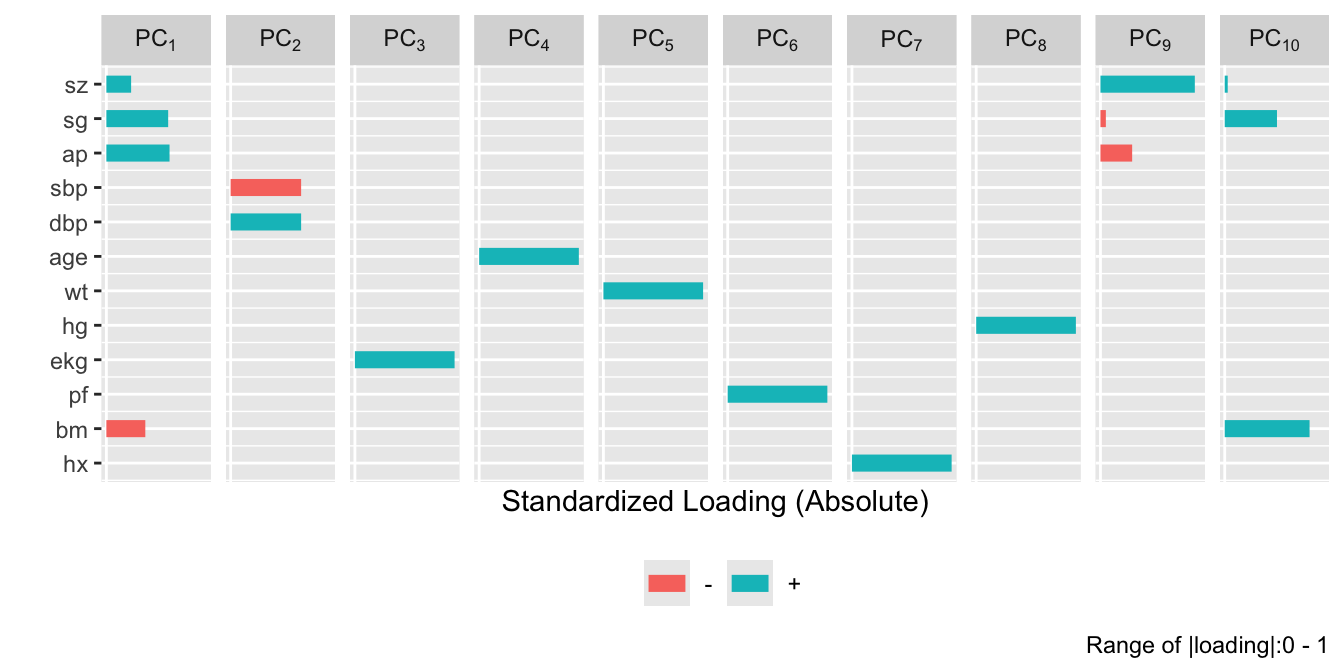Only nonzero loadings are shown. The first sparse PC is the tumor cluster used above, and the second is the blood pressure cluster. Let us see how well incomplete sparse principal component regression predicts time until death. Code spar(bty='l') pcs <- s$scores         # pick off sparse PCs
aic <- numeric(10)
for(i in 1:10) {
ps <- pcs[,1:i]
aic[i] <- AIC(cph(S ~ ps))
}
plot(1:10, aic, xlab='Number of Components Used',
ylab='AIC', type='l',  ylim=c(3950,4000))

More components are required to optimize AIC than were seen in Figure 8.5, but a model built from 6–8 sparse PCs performed as well as the other models.

## 8.7 Transformation Using Nonparametric Smoothers

The ACE nonparametric additive regression method of Breiman & Friedman (1985) transforms both the left-hand-side variable and all the right-hand-side variables so as to optimize $$R^{2}$$. ACE can be used to transform the predictors using the R ace function in the acepack package, called by the transace function in the Hmisc package. transace does not impute data but merely does casewise deletion of missing values. Here transace is run after single imputation by transcan. binary is used to tell transace which variables not to try to predict (because they need no transformation). Several predictors are restricted to be monotonically transformed.

Code
f <- transace(~ monotone(sz) + monotone(sg) + monotone(ap) + monotone(sbp) +
monotone(dbp) + monotone(age) + monotone(pf) + wt + hg +
ekg + bm + hx, data=imputed)
f

Transformations Using Alternating Conditional Expectation

~monotone(sz) + monotone(sg) + monotone(ap) + monotone(sbp) +
monotone(dbp) + monotone(age) + monotone(pf) + wt + hg +
ekg + bm + hx

n= 502

Transformations:

sz          sg          ap         sbp         dbp         age
monotone    monotone    monotone    monotone    monotone    monotone
pf          wt          hg         ekg          bm          hx
monotone     general     general categorical      linear      linear

R-squared achieved in predicting each variable:

sz    sg    ap   sbp   dbp   age    pf    wt    hg   ekg    bm    hx
0.228 0.575 0.573 0.483 0.458 0.152 0.178 0.172 0.202 0.111    NA    NA 
Code
ggplot(f)

Except for ekg, age, and for arbitrary sign reversals, the transformations in Figure 8.8 determined using transace were similar to those in Figure 8.3. The transcan transformation for ekg` makes more sense.

## 8.8 Study Questions

Section 8.2

1. Critique the choice of the number of parameters devoted to each predictor.

Section 8.3

1. Explain why the final amount of redundancy can change from the initial assessment.

Section 8.5

1. What is the meaning of the first canonical variate?
2. Explain the first row of numbers in the matrix of coefficients of canonical variates.
3. The transformations in Figure 8.3 are not optimal for predicting the outcome. What good can be said of them?

Section 8.6

1. Why in general terms do principal components work well in predictive modeling?
2. What is the advantage of sparse PCs over regular PCs?